# 741 IC issue. Still a beginner.

#### Yuseph

Joined Jun 8, 2020
42
Yo guys i have a 741 ic issue.
R2 (at the bottom)= 10kohm
R1 = 1kohm
Differential V - / v+ = 8v
With respect to the gain formula the gain result should be 11.
On the Imput side i have 100mV so on the output i should normally get 1,1V. And im getting 0 instead so what the heck is that.
I changed the ic 3 times. So its not the ic. What am i doing wrong ?

#### AlbertHall

Joined Jun 4, 2014
10,030
It looks like the battery box with no cover is connected between pins 2 and 3 of the IC?
That is not correct.

#### Yuseph

Joined Jun 8, 2020
42
Dude. Negative is connected to pin2 and positive to pin3. Its a noninverting amp. Whats wrong with that.

#### Papabravo

Joined Feb 24, 2006
13,926
Dude. Negative is connected to pin2 and positive to pin3. Its a noninverting amp. Whats wrong with that.
Dude -- those are the inputs. Pins 4 and 7 are the power pins. What is connected to the power pins?
Do you have a schematic drawing, that conveys your intentions? The picture is nearly worthless for that purpose. You need to understand these things when asking for help.

•schmitt trigger

#### Yuseph

Joined Jun 8, 2020
42
Dude -- those are the inputs. Pins 4 and 7 are the power pins. What is connected to the power pins?
Do you have a schematic drawing, that conveys your intentions? The picture is nearly worthless for that purpose. You need to understand these things when asking for help.
You're right.
Pin4 v-
Pin7 v+
Pin2 imput -
Pin3 imput +

#### OBW0549

Joined Mar 2, 2015
3,449
Dude. Negative is connected to pin2 and positive to pin3. Its a noninverting amp. Whats wrong with that.
Dude. You're doing it wrong. You DO NOT connect your input between pins 2 and 3.

The correct way to make a non-inverting amplifier with an op amp is shown right on page 1 of the 741 data sheet. Always read the data sheet.

#### dl324

Joined Mar 30, 2015
10,974
Yo guys i have a 741 ic issue.
R2 (at the bottom)= 10kohm
R1 = 1kohm
Yo dude. How about posting a schematic with the relevant details (like power supply voltages)?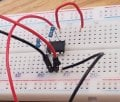I can't see where the hidden terminal of the 10k resistor is going. Looks like it might be pin 1, but that would be a mistake. The feedback resistor is 1k, so you're not going to get a gain of 11.

If you're going to post a 4MB picture, at least make sure it shows relevant details clearly. Then reduce image size for those who have slow internet connections.

What is the battery chemistry? If it's NiMh or NiCd, the circuit can't work because the common mode input range will be essentially nil.

LM741 was a good opamp in it's heyday (best thing since sliced bread at the time), and is still decent for those who know how to use it; but it's not suitable for newbies who can't, or won't, read the datasheet.

#### Yuseph

Joined Jun 8, 2020
42

#### dl324

Joined Mar 30, 2015
10,974
I dont know about the chemistry.
What type of batteries are you using?
R2 is 10kohm and r1 1kohm. That gives 11.
R1 is the feedback resistor. You'd get a gain of 1.1 if you wired it correctly, but R2 is shorted. It seems that you're trying to measure a DC voltage of two of your batteries in series and you're using 2 of those batteries in series as the power source. That don't work. You need to read the datasheet.

The picture you posted has a blue wire that wasn't present in the original picture.
Maybe im not measuring properly.
Your circuit can't work. LM741 doesn't work well with a single supply or bipolar supplies of +/- 2.4V

EDIT: Your original picture shows the input being from a battery holder. That source doesn't have a common ground with the opamp power source. Also, as already mentioned, you don't put your input voltage across both inputs.

Last edited:

#### crutschow

Joined Mar 14, 2008
25,120
The basics:
The V+ and V- power are referenced to ground, not to each other so you need two voltage sources (batteries) with one terminal of each connected to ground.

And each need to be at least 5V for the 741 to operate at all.

Also the input and output can only go to withing about 2V of the supply voltages.

#### twohats

Joined Oct 28, 2015
131
Best use an op-amp designed for a single supply; 0 volts & + volts.
That will work for you.
Good luck..

#### Audioguru again

Joined Oct 21, 2019
1,592
All circuits on the datasheet of a 52 years old 741 opamp have a supply voltage of 30V. Some of them work with only 10V.
Do it like this:

#### sparky 1

Joined Nov 3, 2018
261
checked with the simulation software
The circuit above does work correctly. Changing the voltage Using +/- 8V supply and a gain of 10X or approx 1Vpp output.
R1 should be about 4k. measuring output using multimeter set at AC. The VRMS is then converted to V P-P should be .707

#### Audioguru again

Joined Oct 21, 2019
1,592
checked with the simulation software
The circuit above does work correctly. Changing the voltage Using +/- 8V supply and a gain of 10X or approx 1Vpp output.
R1 should be about 4k. measuring output using multimeter set at AC. The VRMS is then converted to V P-P should be .707

No. If R1 is 4k and R2 is 1k then the voltage gain is 5.0, not 10.
No again. If VRMS is 1.0V then V P-P is 2.828V. If Vpeak is 1.0V then VRMS is 0.707V.

#### Yuseph

Joined Jun 8, 2020
42
All circuits on the datasheet of a 52 years old 741 opamp have a supply voltage of 30V. Some of them work with only 10V.
Do it like this:
Lol i dont even have 6 x 1.5v batteries at my disposal right now. I guess ill postpone to tomorrow.
Thanks for that very clear schematic anyway. Its the fact that v+ and v- were two different supply sources that i missed. Its not drawn like that in my book. And the guys on youtube are long on theory. They skipped the demonstration.
Cant wait to try it.
anyway 3 power sources ?? Why would someone go into so much trouble to amplify voltage ? There must be simplest ways now
And im not sure how i could ever use that on a circuit. Since i tackled the microprocessor topic now.

#### MrChips

Joined Oct 2, 2009
21,331
Is your input signal always positive?
Then you only need a positive power source. You cannot use 741 op-amp for this. You need a rail-to-rail op-amp that can work on a single supply. Something like LTC6261 should work.

#### ci139

Joined Jul 11, 2016
1,559
i recently re-checked the formulas for (img. src. = https://www.maximintegrated.com/en/products/analog/amplifiers/MAX478.html - irrelevant)so i got the calculations (at hand this time)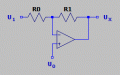U_0=\left({U_X-U_1}\right)·\frac{R_0}{R_0+R_1}+U_1\\ {\ }\\ U_0=U_X·\frac{R_0}{R_0+R_1}-U_1\left({\frac{R_0}{R_0+R_1}-1}\right)\qquad {}^{(1}\\ {\ }\\ U_X=\left[{U_0+U_1\left({\frac{R_0}{R_0+R_1}-1}\right)}\right]·\frac{R_0+R_1}{R_0}\\ {\ }\\ U_X-U_0=U_0\frac{R_0+R_1}{R_0}-U_0+U_1\left({1-\frac{R_0+R_1}{R_0}}\right)\\ {\ }\\ U_X-U_0=U_1\left({1-\frac{R_0+R_1}{R_0}}\right)-U_0\left({1-\frac{R_0+R_1}{R_0}}\right)\\ {\ }\\ \frac{U_X-U_0}{U_1-U_0}=1-\frac{R_0+R_1}{R_0}=\frac{R_0-R_0-R_1}{R_0}=-\frac{R_1}{R_0}\qquad {}^{(2}\\ {\ }\\ \begin{align*}\qquad \qquad \qquad \qquad \qquad \qquad \qquad \qquad \qquad \qquad \qquad \qquad \\ \hline \end{align*}\\ \mathbf{U_1=0}\qquad U_0=U_X·\frac{R_0}{R_0+R_1}\ {}^{from\ the\ (1}\qquad \frac{U_X}{U_0}=\frac{R_0+R_1}{R_0}\\ {\ }\\ \cases{U_X-U_0=\left({-U_0}\right)\left({-\frac{R_1}{R_0}}\right)\ {}^{from\ the\ (2}\\ {\ }\\ U_X=U_0\left({\frac{R_1}{R_0}+1}\right)}\qquad \frac{U_X}{U_0}=\frac{R_0+R_1}{R_0}=1+\frac{R_1}{R_0}

Last edited:

#### ci139

Joined Jul 11, 2016
1,559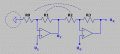$\frac{U_Y}{U_1}=\frac{R_0+R_1}{R_0}\qquad \frac{U_X-U_0}{U_Y-U_0}=-\frac{R_3}{R_2}\\ {\ }\\ \boxed{U_X=}\ U_0\left({\frac{R_2+R_3}{R_2}}\right)-\frac{R_3}{R_2}U_1\left({\frac{R_0+R_1}{R_0}}\right)\ {^?= {}_?}\ \mathbf{...}\ =\left({U_0-U_1}\right)\frac{R_2+R_3}{R_2}\ \boxed{=\Delta U_{IN}\left({1+\mathbf{M}}\right)}\\ {\ }\\ ?\qquad \frac{R_2+R_3}{R_2}=\frac{R_3R_0+R_3R_1}{R_0R_0}\ {^?= {}_?}\ ...\ =\frac{R_3\frac{R_0}{R_1}+R_3}{\frac{R_0}{R_1}R_2}=\frac{R_3\frac{R_3}{R_2}+R_3}{\frac{R_3}{R_2}R_2}=\frac{R_2+R_3}{R_2}\\ {\ }\\ R_0=\frac{R_3\left({R_0+R_1}\right)}{R_3+R_2}\\ {\ }\\ \cases{\underline{R_0R_3}+R_0R_2=\underline{R_0R_3}+R_1R_3\\ {\ }\\ \boxed{R_0R_2=R_1R_3}}\qquad \frac{R_3}{R_2}=\frac{sR_0}{sR_1}=\mathbf{M}\qquad \mathbf{...} \uparrow$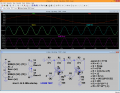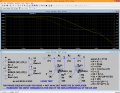High frequency test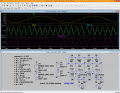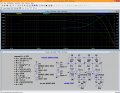Last edited:

#### Audioguru again

Joined Oct 21, 2019
1,592
ANY opamp can is a positive and negative supply, or use just a positive supply if its + input is biased at half the supply voltage and input, output and feedback to ground coupling capacitors are used like this:

#### Attachments

•ci139